Courses

# Vector Spaces & Matrices Physics Notes | EduRev

## Physics : Vector Spaces & Matrices Physics Notes | EduRev

The document Vector Spaces & Matrices Physics Notes | EduRev is a part of the Physics Course Basic Physics for IIT JAM.
All you need of Physics at this link: Physics

Vector Spaces:

Let {\displaystyle F}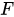be field and let {\displaystyle V}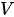be a set. {\displaystyle V}is said to be a Vector Space over {\displaystyle F}along with the binary operations of addition and scalar product if

{\displaystyle \forall \mathbf {A} ,\mathbf {B} ,\mathbf {C} \in V}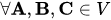(i) {\displaystyle \mathbf {A} +\mathbf {B} =\mathbf {B} +\mathbf {A} }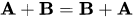...(Commutativity)
(ii) {\displaystyle \mathbf {A} +(\mathbf {B} +\mathbf {C} )=(\mathbf {A} +\mathbf {B} )+\mathbf {C} }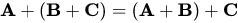...(Associativity)
(iii) {\displaystyle \exists \mathbf {0} \in V}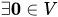such that {\displaystyle \mathbf {A} +\mathbf {0} =\mathbf {A} }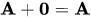...(Identity)
(iv){\displaystyle \exists (\mathbf {-A} )\in V}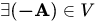such that {\displaystyle \mathbf {A} +\mathbf {-A} =\mathbf {0} }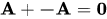...(Inverse)
isplaystyle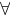{\displaystyle a,b\in F}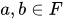(v) {\displaystyle (a+b)\mathbf {A} =a\mathbf {A} +b\mathbf {A} }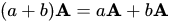(vi){\displaystyle a(\mathbf {A} +\mathbf {B} )=a\mathbf {A} +a\mathbf {B} }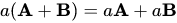(vii){\displaystyle a(b\mathbf {A} )=(ab)\mathbf {A} }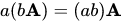The elements of {\displaystyle V}are called vectors while the elements of {\displaystyle F}are called scalars. In most problems of Physics, the field {\displaystyle F}of scalars is either the set of real numbers {\displaystyle \mathbb {R} }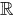or the set of complex numbers {\displaystyle \mathbb {C} }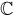.
Examples of vector spaces:
(i) The set {\displaystyle \mathbb {R} ^{n}}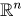over {\displaystyle \mathbb {R} }can be visualised as the space of ordinary vectors "arrows" of elementary Physics.
(ii) The set of all real polynomials {\displaystyle P(x)=a_{0}+a_{1}x+\ldots +a_{n}x^{n}}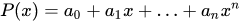is a vector space over {\displaystyle \mathbb {R} }(iii) Indeed, the set of all functions {\displaystyle f:[a,b]\to \mathbb {R} }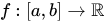is also a vector spaces over {\displaystyle \mathbb {R} }, with addition and scalar multiplication defined as is usual.
Although the idea of vectors as "arrows" works well in most examples of vector spaces and is useful in solving problems, the latter two examples were deliberately provided as cases where this intuition fails to work.

Basis:

A set E ⊂ V is said to be linearly independent if and only if
a1E+ a2E2 + ... + anEn =0 implies that a1 =a= ... =0, whenever E1, E2,....En ∈ E

A set E ⊂ V is said to cover V{\displaystyle V} if for every A ∈ V there exist e1,e2,... such that A = e1E1 + e2E+ ... (we leave the question of finiteness of the number of terms open at this point)

A set E ⊂ V is said to be a basis for V if B is linearly independent and if covers V

If a vector space has a finite basis with n{\displaystyle n} elements, the vetor space is said to be n-dimensional

As an example, we can consider the vector space R3{\displaystyle \mathbb {R} ^{3}} over reals. The vectors (1,0,0); (0,1,0); (0,0,1){\displaystyle (1,0,0);(0,1,0);(0,0,1)} form one of the several possible basis for R3{\displaystyle \mathbb {R} ^{3}}. These vectors are often denoted as {\displaystyle {\hat {i}},{\hat {j}},{\hat {k}}}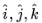or as {\displaystyle {\hat {x}},{\hat {y}},{\hat {z}}}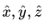Theorem:

Let V{\displaystyle V} be a vector space and let B={b1,b2,...,bn}{\displaystyle B=\{\mathbf {b} _{1},\mathbf {b} _{2},\ldots ,\mathbf {b} _{n}\}} be a basis for V{\displaystyle V}. Then any subset of V{\displaystyle V} with n+1{\displaystyle n+1} elements is linearly dependent.

Proof :
Let E ⊂ V with E = {u1,u2,..,un+1}
By definition of basis, there exist scalars a1i,a2i,...,ani such that ui =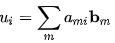{\displaystyle B\subset V}{\displaystyle e_{1},e_{2},\ldots }Hence we can write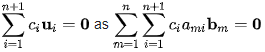that is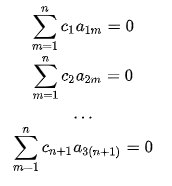Which has a nontrivial solution for ci{\displaystyle c_{i}}. Hence E{\displaystyle E} is linearly dependent.
If a vector space has a finite basis of n{\displaystyle n} elements, we say that the vector space is n-dimensional
Inner Product:

An in-depth treatment of inner-product spaces will be provided in the chapter on Hilbert Spaces. Here we wish to provide an introduction to the inner product using a basis.
Let V{\displaystyle V} be a vector space over R{\displaystyle \mathbb {R} } and let B={b1,b2,...,bnbe a basis for V{\displaystyle V}Thus for every member u{\displaystyle \mathbf {u} } of V{\displaystyle V}we can write {\displaystyle \mathbf {u} =\displaystyle \sum _{i=1}^{n}b_{i}\mathbf {b} _{i}}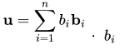{\displaystyle b_{ are called the components of {\displaystyle \mathbf {u} }with respect to the basis B{\displaystyle B}.

We define the inner product as a binary operation (.) : V x V → R {\displaystyle (\cdot ):V\times V\to \mathbb {R} } as {\displaystyle \mathbf {x} \cdot \mathbf {y} =\displaystyle \sum _{i}x_{i}y_{i}}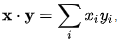where xi,yi{\displaystyle x_{i},y_{i}} are the components of x,y{\displaystyle \mathbf {x} ,\mathbf {y} } with respect to B

Note here that the inner product so defined is intrinsically dependent on the basis. Unless otherwise mentioned, we will assume the basis {\displaystyle {\hat {x}},{\hat {y}},{\hat {z}}}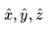while dealing with inner product of ordinary "vectors".

Linear Transformations:

Let U{\displaystyle U}, V{\displaystyle be vector spaces over F{\displaystyle F}A function T : U → V{\displaystyle T:U\to V} is said to be a Linear transformation if for all u1, u2{\displaystyle \mathbf {u} _{1},\mathbf {u} _{2}\in U ∈ U and c ∈ F{\displaystyle c\in F} if
(i)T(u1 + u2) = T(u1) + T(u2)
(ii)T(cu1) = cT(u1)
Now let E = {e1,e2,...,em}{\displaystyle E=\{\mathbf {e} _{1},\mathbf {e} _{2},\ldots ,\mathbf {e} _{m}\}} and F = {f1,f2,...,fn}{\displaystyle F=\{\mathbf {f} _{1},\mathbf {f} _{2},\ldots ,\mathbf {f} _{n}\}} be bases for U,V{\displaystyle U,V} respectively.
Let e′=T(e1).{\displaystyle \mathbf {e'} _{i}=T(\mathbf {e} _{i}) As F{\displaystyle F} is a basis, we can write {\displaystyle \mathbf {e'} _{i}=\sum _{j}a_{ij}\mathbf {f} _{j}}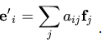Thus, by linearity we can say that if T(u) =vplaystyle T(\mathbf {u} )=\mathbf {v} }, we can write the components uj{\displaystyle v_{j}} of v{\displaystyle \mathbf {v} } in terms of those of u{\displaystyle \mathbf {u} } as {\displaystyle v_{j}=\sum _{i}u_{i}a_{ij}}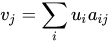The collection of coefficients aij{\displaystyle a_{ij}} is called a matrix, written as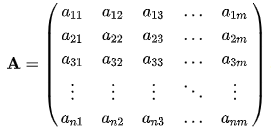{\displaystyle T(c\mathbf {u} _{1})=cT(\mathbf {u} _{1})}and we can say that T{\displaystyle T} can be represented as a matrix A{\displaystyle \mathbf {A} } with respect to the bases E,F{\displaystyle E,F}

Eigenvalue Problems:

Let V{\displaystyle V} be a vector space over reals and let T : V → V{\displaystyle T:V\to V} be a linear transformations.

Equations of the type Tu = λu{\displaystyle T\mathbf {u} =\lambda \mathbf {u} }, to be solved for u ∈ V{\displaystyle \mathbf {u} \in V} and λ ∈ R{\displaystyle \lambda \in \mathbb {R} } are called eigenvalue problems. The solutions {\displaystyle \lambda }λ are called eigenvalues of T{\displaystyle T} while the corresponding u{\displaystyle \mathbf {u} } are called eigenvectors or eigenfunctions. (Here we take Tu = T(u){\displaystyle T\mathbf {u} =T(\mathbf {u} )} )

Matrices

Definition:

Let F                    F              {\displaystyle F}    be a field and let M = {1,2,...,m}              M        =        {        1        ,        2        ,        …        ,        m        }              {\displaystyle M=\{1,2,\ldots ,m\}}   , N = {1,2,...n}                    N        =        {        1        ,        2        ,        …        ,        n        }              {\displaystyle N=\{1,2,\ldots ,n\}}   . An n×m matrix is a function A :N x M → F                  A        :        N        ×        M        →        F              {\displaystyle A:N\times M\to F}

We denote A(ij) = aij                    A        (        i        ,        j        )        =                  a                      i            j                                {\displaystyle A(i,j)=a_{ij}}   . Thus, the matrix A                     A              {\displaystyle A}   can be written as the array of numbers A =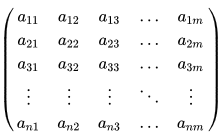A        =                              (                                                                                a                                          11                                                                                                            a                                          12                                                                                                            a                                          13                                                                                        …                                                                      a                                          1                      m                                                                                                                                        a                                          21                                                                                                            a                                          22                                                                                                            a                                          23                                                                                        …                                                                      a                                          2                      m                                                                                                                                        a                                          31                                                                                                            a                                          32                                                                                                            a                                          33                                                                                        …                                                                      a                                          3                      m                                                                                                                    ⋮                                                  ⋮                                                  ⋮                                                  ⋱                                                  ⋮                                                                                                  a                                          n                      1                                                                                                            a                                          n                      2                                                                                                            a                                          n                      3                                                                                        …                                                                      a                                          n                      m                                                                                            )                                {\displaystyle A={\begin{pmatrix}a_{11}&a_{12}&a_{13}&\ldots &a_{1m}\\a_{21}&a_{22}&a_{23}&\ldots &a_{2m}\\a_{31}&a_{32}&a_{33}&\ldots &a_{3m}\\\vdots &\vdots &\vdots &\ddots &\vdots \\a_{n1}&a_{n2}&a_{n3}&\ldots &a_{nm}\\\end{pmatrix}}}

Consider the set of all n×m matrices defined on a field F                    F              {\displaystyle F}   . Let us define scalar product cA                     c        A              {\displaystyle cA}   to be the matrix B                    B              {\displaystyle B}    whose elements are given by bij = caij                              b                      i            j                          =        c                  a                      i            j                                {\displaystyle b_{ij}=ca_{ij}}   . Also let addition of two matrices A + B                    A        +        B              {\displaystyle A+B}    be the matrix C                     C              {\displaystyle C}   whose elements are given by cij = aij + bij                               c                      i            j                          =                  a                      i            j                          +                  b                      i            j                                {\displaystyle c_{ij}=a_{ij}+b_{ij}}
With these definitions, we can see that the set of all n×m matrices on F                    F              {\displaystyle F}    form a vector space over F
Linear Transformations:

Let U, V be vector spaces over the field F Consider the set of all linear transformations T : U → V .

Define addition of transformations as (T1+ T2 ) 1 1 = T1u - T2u and scalar product as (CT)u =c(Tu). Thus, the set of all linear transformations from U to V is a vector space. This space is denoted as L(U, V).

Observe that L(U, V) is an run dimensional vector space
Operations on Matrices:

Determinant:

The determinant of a matrix is defined iteratively (a determinant can be defined only if the matrix is square). If A is a matrix, its determinant is denoted as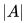We define ,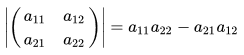For n=3                    n        =        3              {\displaystyle n=3}   , we define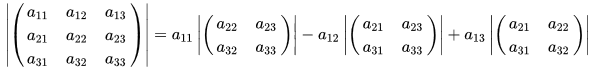we thus define the determinant for any square matrix

Trace:

Let A                    A              {\displaystyle A}    be an n×n (square) matrix with elements                               a                      i            j                                {\displaystyle a_{ij}}   aij

The trace of A                    A              {\displaystyle A}    is defined as the sum of its diagonal elements, that is,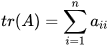This is conventionally denoted as                     t        r        (        A        )        =                  ∑                      i            ,            j            =            1                                n                                    a                      i            j                                    δ                      i            j                                {\displaystyle tr(A)=\sum _{i,j=1}^{n}a_{ij}\delta _{ij}}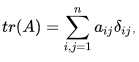where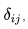called the Kronecker delta is a symbol which you will encounter constantly in this book. It is defined as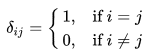The Kronecker delta itself denotes the members of an n×n matrix called the n×n unit matrix, denoted as                     I              {\displaystyle I}    I

Transpose:

Let A                    A              {\displaystyle A}    be an m×n matrix, with elements                           a                      i            j                                {\displaystyle a_{ij}}   aij. The n×m matrix AT                              A                      T                                {\displaystyle A^{T}}    with elements                               a                      i            j                                T                                {\displaystyle a_{ij}^{T}}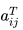is called the transpose of A                    A              {\displaystyle A}    when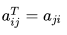a                      i            j                                T                          =                  a                      j            i                                {\displaystyle a_{ij}^{T}=a_{ji}}

Matrix Product:

Let A                    A              {\displaystyle A}    be an m×n matrix and let B                    B              {\displaystyle B}    be an n×p matrix.

We define the product of A,B                    A        ,        B              {\displaystyle A,B}    to be the m×p matrix C                    C              {\displaystyle C}    whose elements are given by

F              {\displaystyle F}

aystyle E\subset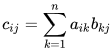and we write                     C        =        A        B              {\displaystyle C=AB}    C =AB

Properties:

(i) Product of matrices is not commutative. Indeed, for two matrices A,B                    A        ,        B              {\displaystyle A,B}   , the product BA                   B        A              {\displaystyle BA}    need not be well-defined even though AB                    A        B              {\displaystyle AB}    can be defined as above.
(ii) For any matrix n×n A we have AI = IA = A, where I is the n×n unit matrix.

Offer running on EduRev: Apply code STAYHOME200 to get INR 200 off on our premium plan EduRev Infinity!

,

,

,

,

,

,

,

,

,

,

,

,

,

,

,

,

,

,

,

,

,

;